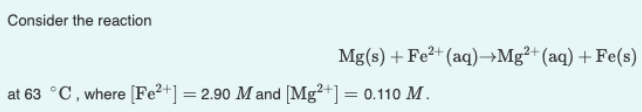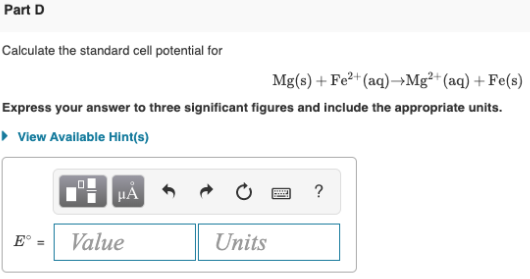# Consider the reaction Mg(s) + Fe^2+(aq) → Mg^2+(aq) + Fe(s) at 63 °C, where [Fe62+] = 2.90 M and [Mg62+] = 0.110 M. Calculate the standard cell potential for Mg(s) + Fe^2+(aq) → Mg^2+(aq) + Fe(s) Express your answer to three significant figures and include the appropriate units.